# MLflow Model Registry example

This example illustrates how to use MLflow Model Registry to build a machine learning application that forecasts the daily power output of a wind farm. The example shows how to:

• Track and log models with MLflow

• Register models with the Model Registry

• Describe models and make model version stage transitions

• Integrate registered models with production applications

• Search and discover models in the Model Registry

• Archive and delete models

The article describes how to perform these steps using the MLflow Tracking and MLflow Model Registry UIs and APIs.

For a notebook that performs all these steps using the MLflow Tracking and Registry APIs, see the Model Registry example notebook.

## Load dataset, train model, and track with MLflow Tracking

Before you can register a model in the Model Registry, you must first train and log the model during an experiment run. This section shows how to load the wind farm dataset, train a model, and log the training run to MLflow.

The following code loads a dataset containing weather data and power output information for a wind farm in the United States. The dataset contains `wind direction`, `wind speed`, and `air temperature` features sampled every six hours (once at `00:00`, once at `08:00`, and once at `16:00`), as well as daily aggregate power output (`power`), over several years.

```import pandas as pd

def get_training_data():
training_data = pd.DataFrame(wind_farm_data["2014-01-01":"2018-01-01"])
X = training_data.drop(columns="power")
y = training_data["power"]
return X, y

def get_validation_data():
validation_data = pd.DataFrame(wind_farm_data["2018-01-01":"2019-01-01"])
X = validation_data.drop(columns="power")
y = validation_data["power"]
return X, y

def get_weather_and_forecast():
format_date = lambda pd_date : pd_date.date().strftime("%Y-%m-%d")
today = pd.Timestamp('today').normalize()
week_ago = today - pd.Timedelta(days=5)
week_later = today + pd.Timedelta(days=5)

past_power_output = pd.DataFrame(wind_farm_data)[format_date(week_ago):format_date(today)]
weather_and_forecast = pd.DataFrame(wind_farm_data)[format_date(week_ago):format_date(week_later)]
if len(weather_and_forecast) < 10:
past_power_output = pd.DataFrame(wind_farm_data).iloc[-10:-5]
weather_and_forecast = pd.DataFrame(wind_farm_data).iloc[-10:]

return weather_and_forecast.drop(columns="power"), past_power_output["power"]
```

### Train model

The following code trains a neural network using TensorFlow Keras to predict power output based on the weather features in the dataset. MLflow is used to track the model’s hyperparameters, performance metrics, source code, and artifacts.

```def train_keras_model(X, y):
import tensorflow.keras
from tensorflow.keras.models import Sequential
from tensorflow.keras.layers import Dense

model = Sequential()

model.fit(X_train, y_train, epochs=100, batch_size=64, validation_split=.2)
return model

import mlflow

X_train, y_train = get_training_data()

with mlflow.start_run():
# Automatically capture the model's parameters, metrics, artifacts,
# and source code with the `autolog()` function
mlflow.tensorflow.autolog()

train_keras_model(X_train, y_train)
run_id = mlflow.active_run().info.run_id
```

## Register and manage the model using the MLflow UI

### Create a new registered model

1. Navigate to the MLflow Experiment Runs sidebar by clicking the Experiment iconin the Databricks notebook’s right sidebar.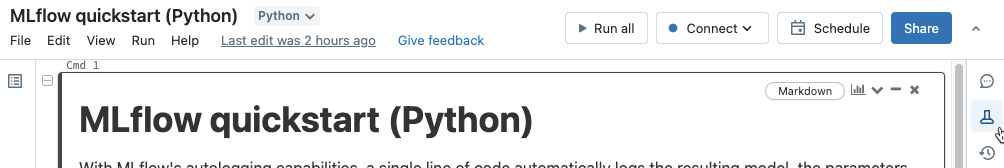2. Locate the MLflow Run corresponding to the TensorFlow Keras model training session, and open it in the MLflow Run UI by clicking the View Run Detail icon.

3. In the MLflow UI, scroll down to the Artifacts section and click the directory named model. Click the Register Model button that appears.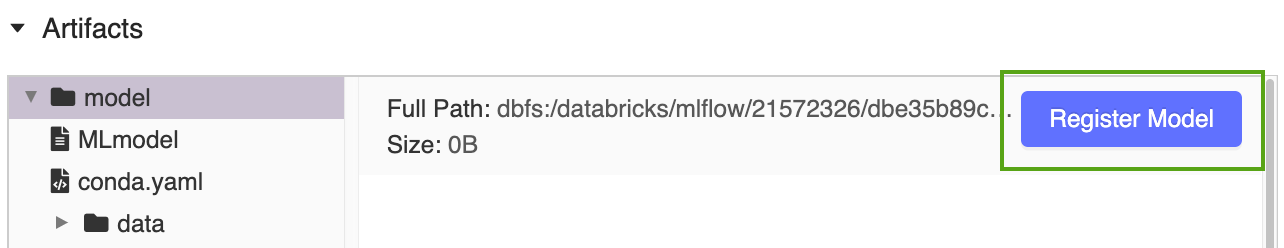4. Select Create New Model from the drop-down menu, and input the following model name: `power-forecasting-model`.

5. Click Register. This registers a new model called `power-forecasting-model` and creates a new model version: `Version 1`.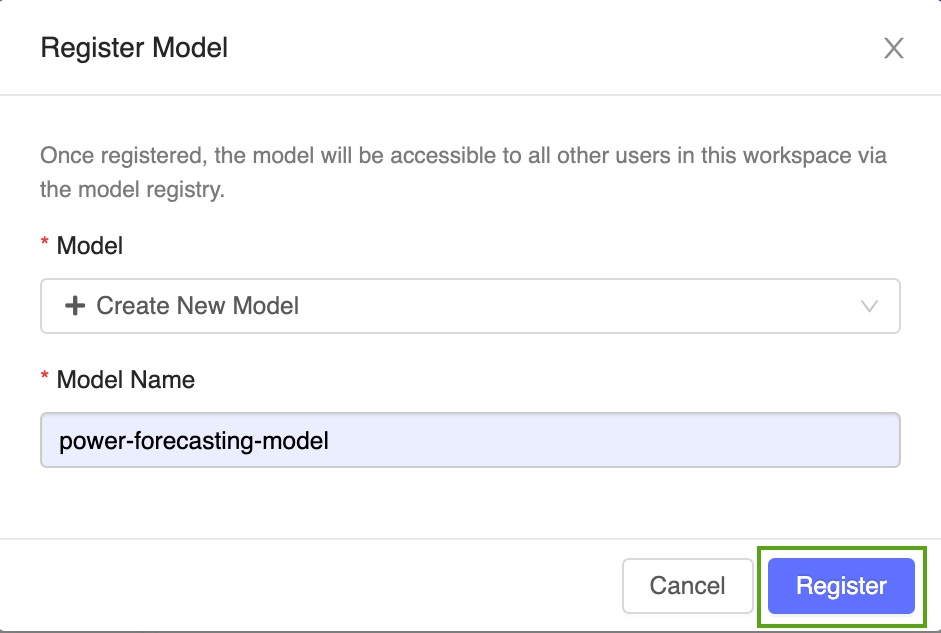After a few moments, the MLflow UI displays a link to the new registered model. Follow this link to open the new model version in the MLflow Model Registry UI.

### Explore the Model Registry UI

The model version page in the MLflow Model Registry UI provides information about `Version 1` of the registered forecasting model, including its author, creation time, and its current stage.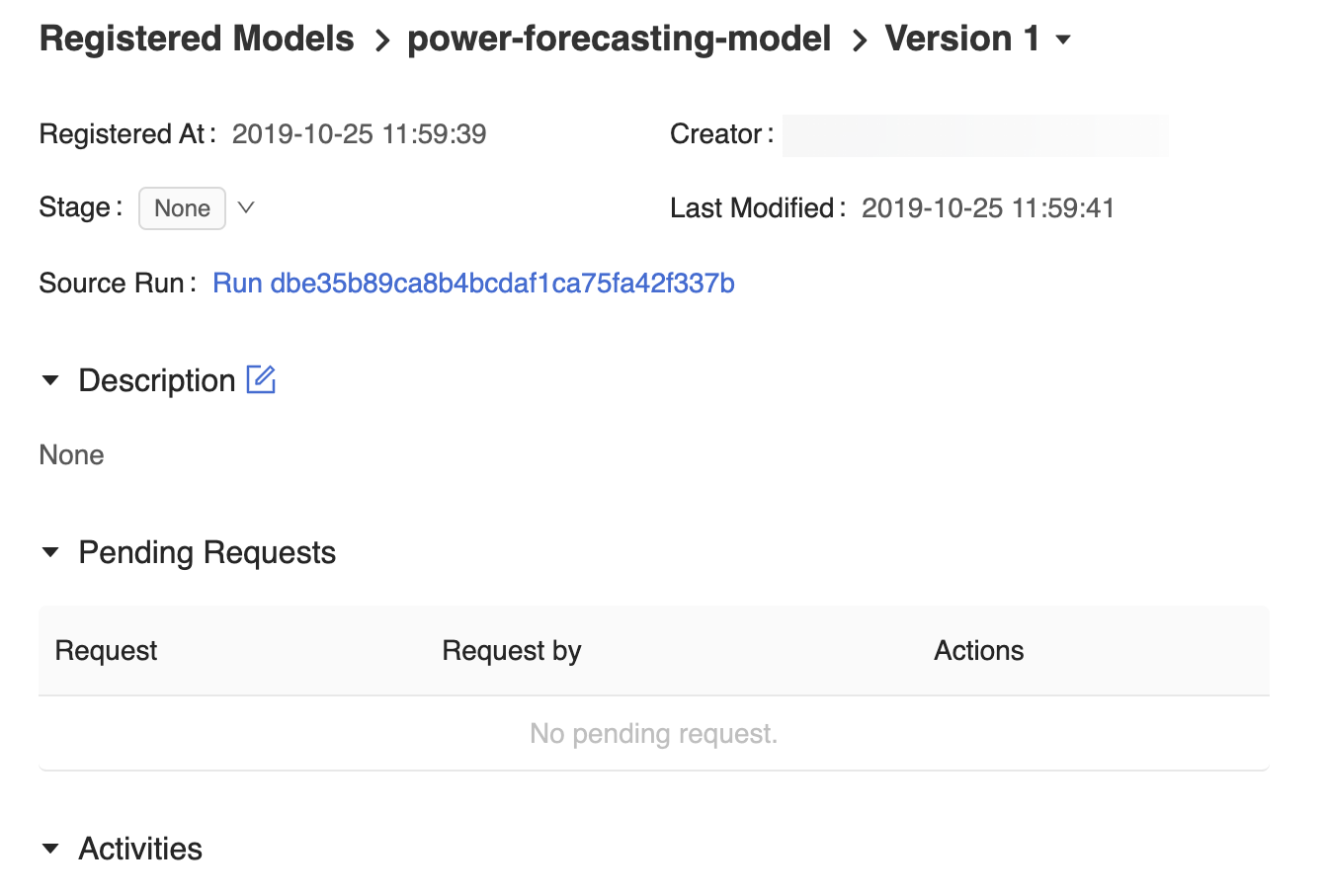The model version page also provides a Source Run link, which opens the MLflow Run that was used to create the model in the MLflow Run UI. From the MLflow Run UI, you can access the Source notebook link to view a snapshot of the Databricks notebook that was used to train the model.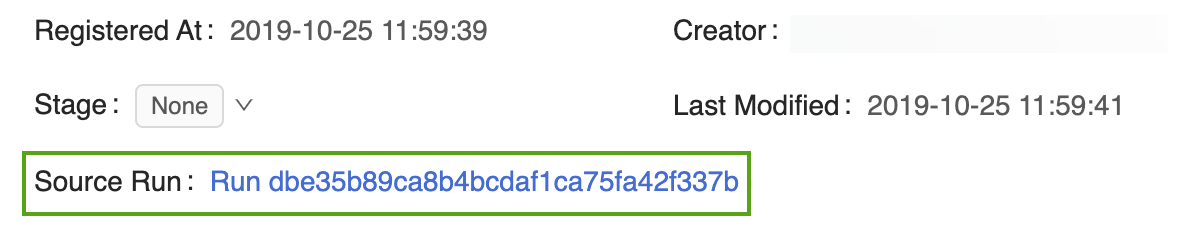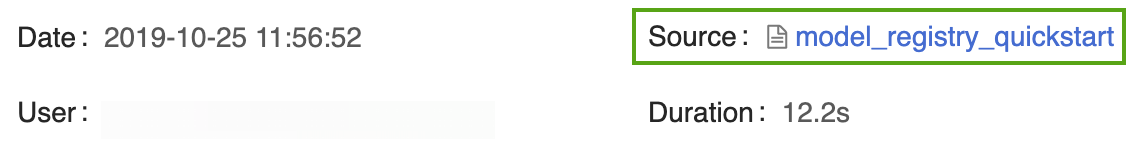To navigate back to the MLflow Model Registry, click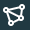Models in sidebar.

The resulting MLflow Model Registry home page displays a list of all the registered models in your Databricks workspace, including their versions and stages.

Click the power-forecasting-model link to open the registered model page, which displays all of the versions of the forecasting model.

You can add descriptions to registered models and model versions. Registered model descriptions are useful for recording information that applies to multiple model versions (e.g., a general overview of the modeling problem and dataset). Model version descriptions are useful for detailing the unique attributes of a particular model version (e.g., the methodology and algorithm used to develop the model).

1. Add a high-level description to the registered power forecasting model. Click the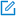icon and enter the following description:

```This model forecasts the power output of a wind farm based on weather data. The weather data consists of three features: wind speed, wind direction, and air temperature.
```2. Click Save.

3. Click the Version 1 link from the registered model page to navigate back to the model version page.

4. Click theicon and enter the following description:

```This model version was built using TensorFlow Keras. It is a feed-forward neural network with one hidden layer.
```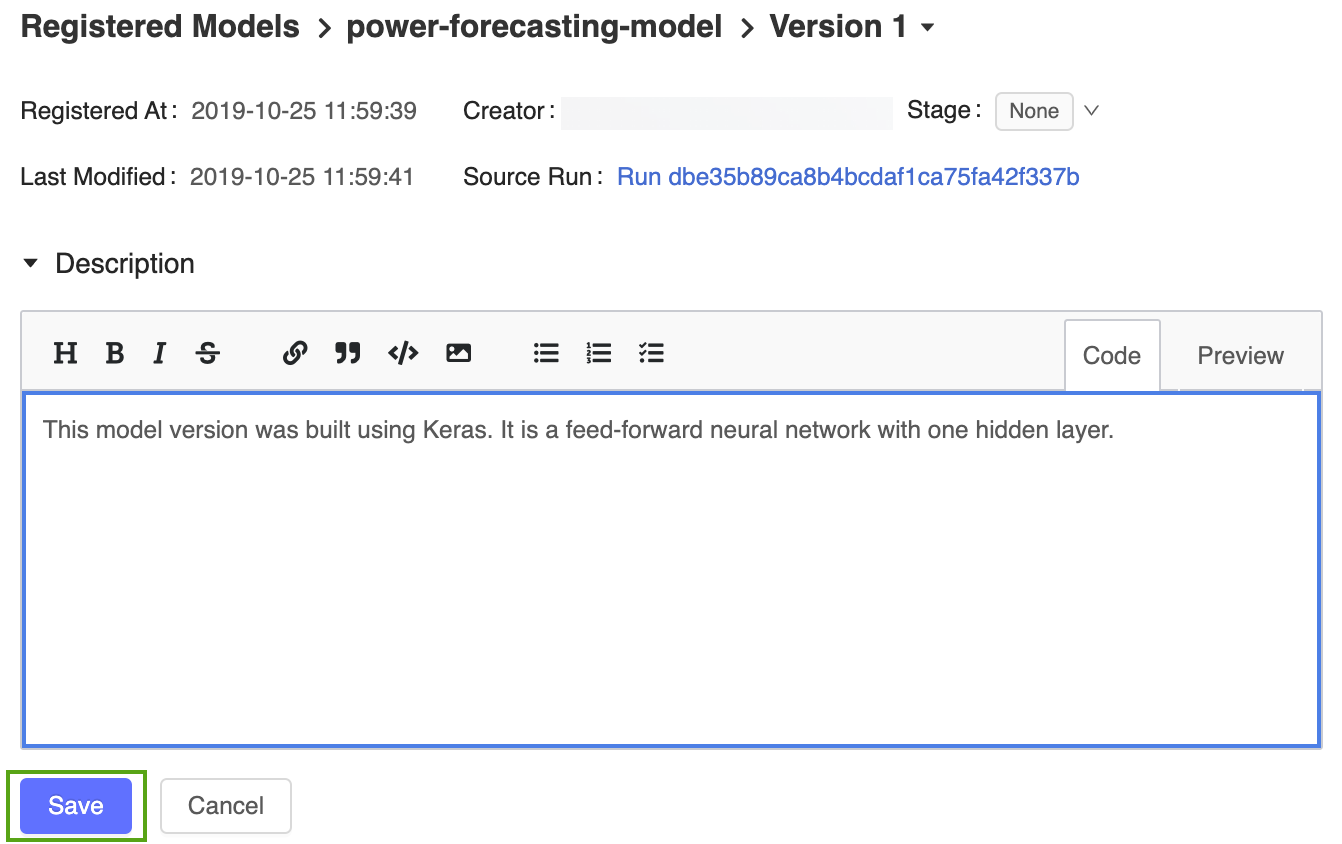5. Click Save.

### Transition a model version

The MLflow Model Registry defines several model stages: None, Staging, Production, and `Archived`. Each stage has a unique meaning. For example, Staging is meant for model testing, while Production is for models that have completed the testing or review processes and have been deployed to applications.

1. Click the Stage button to display the list of available model stages and your available stage transition options.

2. Select Transition to -> Production and press OK in the stage transition confirmation window to transition the model to Production.After the model version is transitioned to Production, the current stage is displayed in the UI, and an entry is added to the activity log to reflect the transition.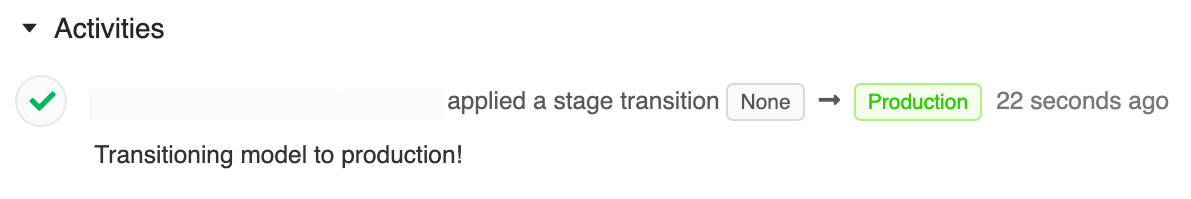The MLflow Model Registry allows multiple model versions to share the same stage. When referencing a model by stage, the Model Registry uses the latest model version (the model version with the largest version ID). The registered model page displays all of the versions of a particular model.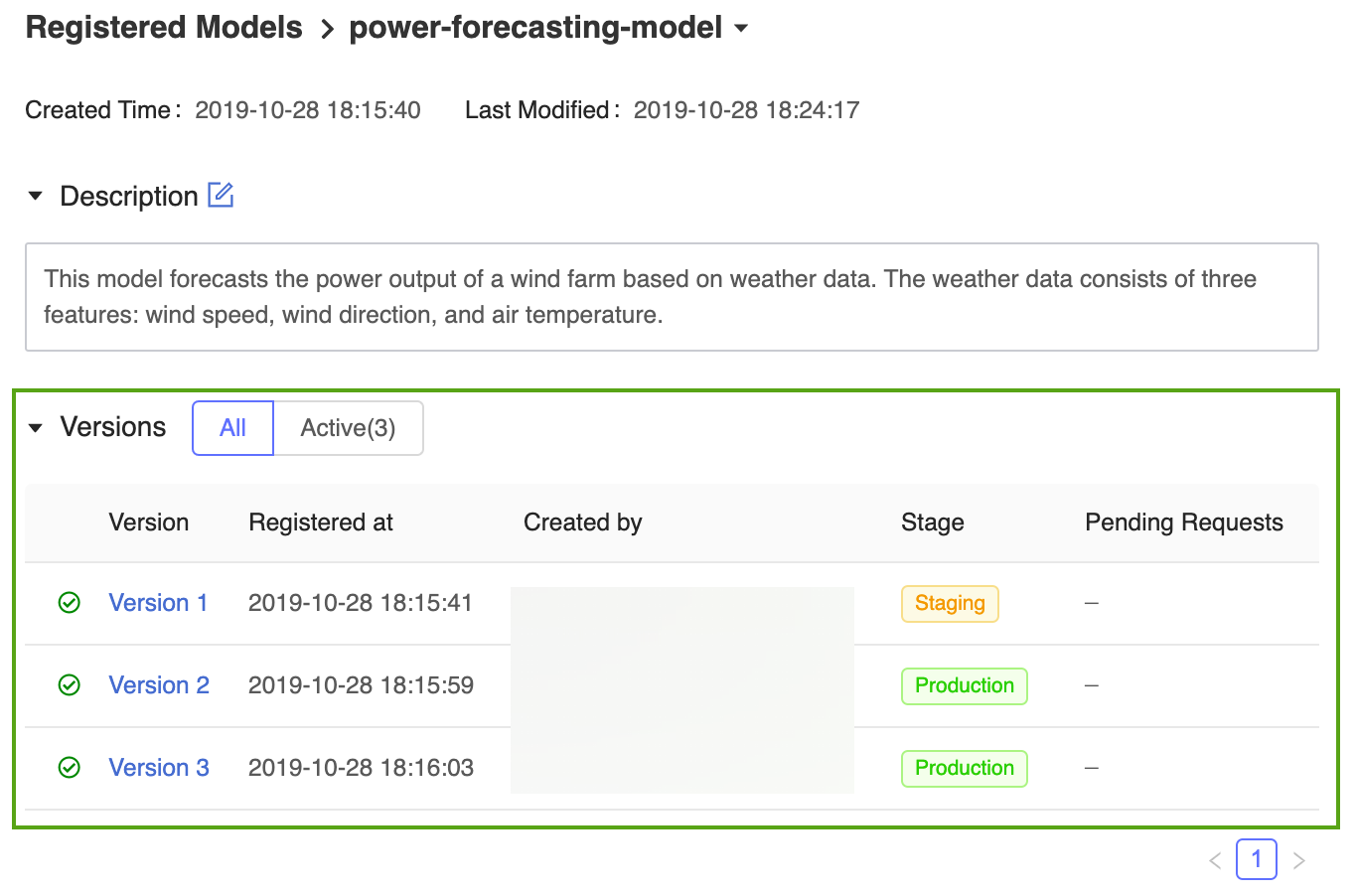## Register and manage the model using the MLflow API

### Define the model’s name programmatically

Now that the model has been registered and transitioned to Production, you can reference it using MLflow programmatic APIs. Define the registered model’s name as follows:

```model_name = "power-forecasting-model"
```

### Register the model

```model_name = get_model_name()

import mlflow

# The default path where the MLflow autologging function stores the TensorFlow Keras model
artifact_path = "model"
model_uri = "runs:/{run_id}/{artifact_path}".format(run_id=run_id, artifact_path=artifact_path)

model_details = mlflow.register_model(model_uri=model_uri, name=model_name)

import time
from mlflow.tracking.client import MlflowClient
from mlflow.entities.model_registry.model_version_status import ModelVersionStatus

# Wait until the model is ready
client = MlflowClient()
for _ in range(10):
model_version_details = client.get_model_version(
name=model_name,
version=model_version,
)
status = ModelVersionStatus.from_string(model_version_details.status)
print("Model status: %s" % ModelVersionStatus.to_string(status))
break
time.sleep(1)

```

### Add model and model version descriptions using the API

```from mlflow.tracking.client import MlflowClient

client = MlflowClient()
client.update_registered_model(
name=model_details.name,
description="This model forecasts the power output of a wind farm based on weather data. The weather data consists of three features: wind speed, wind direction, and air temperature."
)

client.update_model_version(
name=model_details.name,
version=model_details.version,
description="This model version was built using TensorFlow Keras. It is a feed-forward neural network with one hidden layer."
)
```

### Transition a model version and retrieve details using the API

```client.transition_model_version_stage(
name=model_details.name,
version=model_details.version,
stage='production',
)
model_version_details = client.get_model_version(
name=model_details.name,
version=model_details.version,
)
print("The current model stage is: '{stage}'".format(stage=model_version_details.current_stage))

latest_version_info = client.get_latest_versions(model_name, stages=["production"])
latest_production_version = latest_version_info.version
print("The latest production version of the model '%s' is '%s'." % (model_name, latest_production_version))
```

## Load versions of the registered model using the API

The MLflow Models component defines functions for loading models from several machine learning frameworks. For example, `mlflow.tensorflow.load_model()` is used to load TensorFlow models that were saved in MLflow format, and `mlflow.sklearn.load_model()` is used to load scikit-learn models that were saved in MLflow format.

These functions can load models from the MLflow Model Registry.

```import mlflow.pyfunc

model_version_uri = "models:/{model_name}/1".format(model_name=model_name)

model_production_uri = "models:/{model_name}/production".format(model_name=model_name)

```

## Forecast power output with the production model

In this section, the production model is used to evaluate weather forecast data for the wind farm. The `forecast_power()` application loads the latest version of the forecasting model from the specified stage and uses it to forecast power production over the next five days.

```def plot(model_name, model_stage, model_version, power_predictions, past_power_output):
import pandas as pd
import matplotlib.dates as mdates
from matplotlib import pyplot as plt
index = power_predictions.index
fig = plt.figure(figsize=(11, 7))
ax.tick_params(axis='both', which='major', labelsize=17)
ax.xaxis.set_major_formatter(mdates.DateFormatter('%m/%d'))
ax.plot(index[:len(past_power_output)], past_power_output, label="True", color="red", alpha=0.5, linewidth=4)
ax.plot(index, power_predictions.squeeze(), "--", label="Predicted by '%s'\nin stage '%s' (Version %d)" % (model_name, model_stage, model_version), color="blue", linewidth=3)
ax.set_ylim(ymin=0, ymax=max(3500, int(max(power_predictions.values) * 1.3)))
ax.legend(fontsize=14)
plt.title("Wind farm power output and projections", size=24, pad=20)
plt.tight_layout()
display(plt.show())

def forecast_power(model_name, model_stage):
from mlflow.tracking.client import MlflowClient
client = MlflowClient()
model_version = client.get_latest_versions(model_name, stages=[model_stage]).version
model_uri = "models:/{model_name}/{model_stage}".format(model_name=model_name, model_stage=model_stage)
weather_data, past_power_output = get_weather_and_forecast()
power_predictions = pd.DataFrame(model.predict(weather_data))
power_predictions.index = pd.to_datetime(weather_data.index)
print(power_predictions)
plot(model_name, model_stage, int(model_version), power_predictions, past_power_output)
```

## Create a new model version

Classical machine learning techniques are also effective for power forecasting. The following code trains a random forest model using scikit-learn and registers it with the MLflow Model Registry via the `mlflow.sklearn.log_model()` function.

```import mlflow.sklearn
from sklearn.ensemble import RandomForestRegressor
from sklearn.metrics import mean_squared_error

with mlflow.start_run():
n_estimators = 300
mlflow.log_param("n_estimators", n_estimators)

rand_forest = RandomForestRegressor(n_estimators=n_estimators)
rand_forest.fit(X_train, y_train)

val_x, val_y = get_validation_data()
mse = mean_squared_error(rand_forest.predict(val_x), val_y)
print("Validation MSE: %d" % mse)
mlflow.log_metric("mse", mse)

# Specify the `registered_model_name` parameter of the `mlflow.sklearn.log_model()`
# function to register the model with the MLflow Model Registry. This automatically
# creates a new model version
mlflow.sklearn.log_model(
sk_model=rand_forest,
artifact_path="sklearn-model",
registered_model_name=model_name,
)
```

### Add a description to the new model version

```client.update_model_version(
name=model_name,
version=new_model_version,
description="This model version is a random forest containing 100 decision trees that was trained in scikit-learn."
)
```

### Transition the new model version to Staging and test the model

Before deploying a model to a production application, it is often best practice to test it in a staging environment. The following code transitions the new model version to Staging and evaluates its performance.

```client.transition_model_version_stage(
name=model_name,
version=new_model_version,
stage="Staging",
)

forecast_power(model_name, "Staging")
```

### Deploy the new model version to Production

After verifying that the new model version performs well in staging, the following code transitions the model to Production and uses the exact same application code from the Forecast power output with the production model section to produce a power forecast.

```client.transition_model_version_stage(
name=model_name,
version=new_model_version,
stage="production",
)

forecast_power(model_name, "production")
```

There are now two model versions of the forecasting model in the Production stage: the model version trained in Keras model and the version trained in scikit-learn.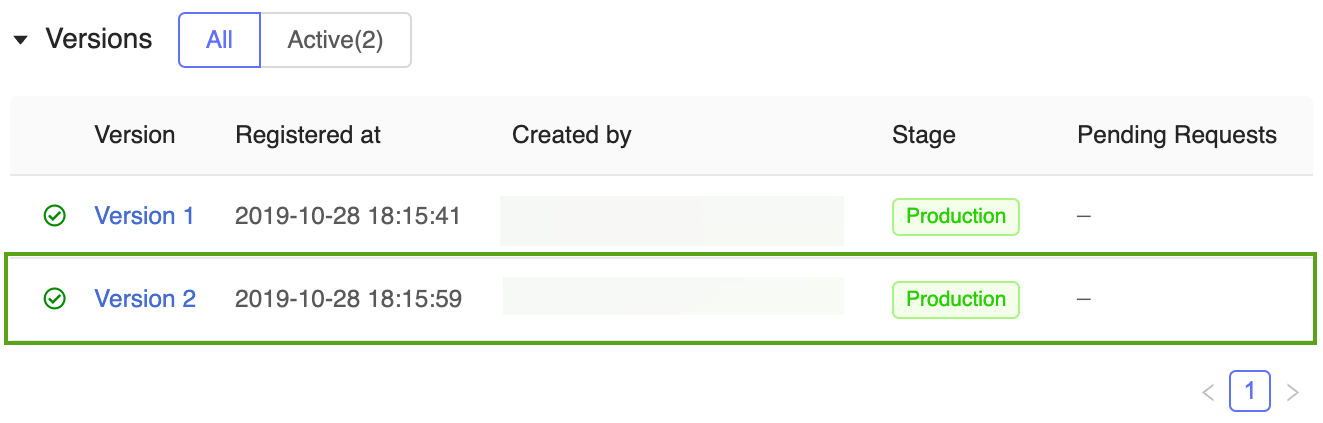Note

When referencing a model by stage, the MLflow Model Model Registry automatically uses the latest production version. This enables you to update your production models without changing any application code.

## Archive and delete models

When a model version is no longer being used, you can archive it or delete it. You can also delete an entire registered model; this removes all of its associated model versions.

### Archive `Version 1` of the power forecasting model

Archive `Version 1` of the power forecasting model because it is no longer being used. You can archive models in the MLflow Model Registry UI or via the MLflow API.

### Archive `Version 1` in the MLflow UI

To archive `Version 1` of the power forecasting model:

1. Open its corresponding model version page in the MLflow Model Registry UI:2. Click the Stage button, select Transition To -> Archived: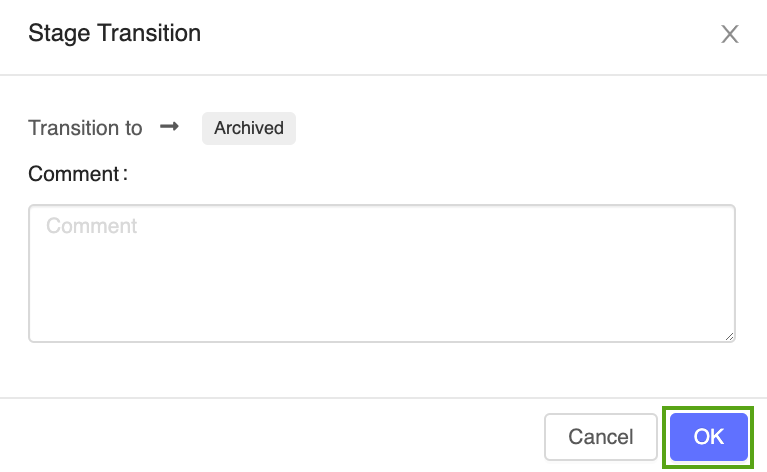3. Press OK in the stage transition confirmation window.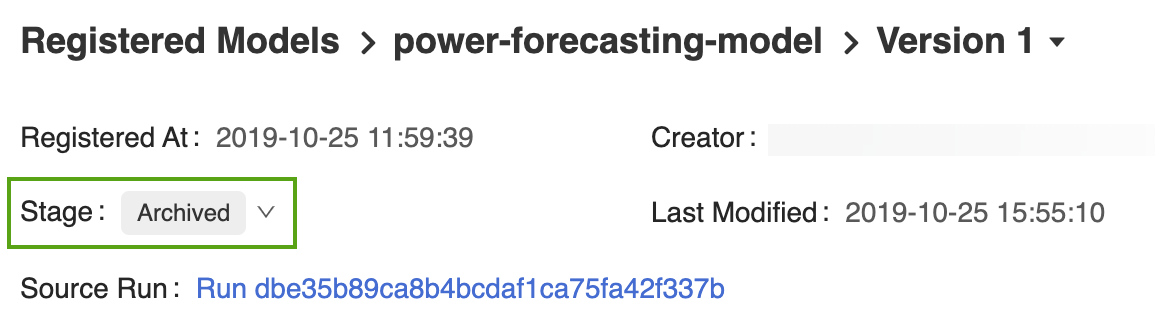#### Archive `Version 1` using the MLflow API

The following code uses the `MlflowClient.update_model_version()` function to archive `Version 1` of the power forecasting model.

```from mlflow.tracking.client import MlflowClient

client = MlflowClient()
client.transition_model_version_stage(
name=model_name,
version=1,
stage="Archived",
)
```

#### Delete `Version 1` of the power forecasting model

You can also use the MLflow UI or MLflow API to delete model versions.

Warning

Model version deletion is permanent and cannot be undone.

##### Delete `Version 1` in the MLflow UI

To delete `Version 1` of the power forecasting model:

1. Open its corresponding model version page in the MLflow Model Registry UI.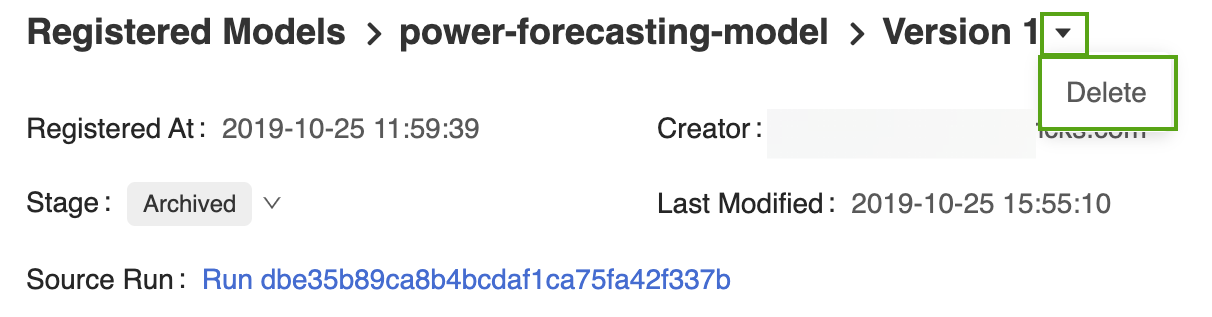2. Select the drop-down arrow next to the version identifier and click Delete.

##### Delete `Version 1` using the MLflow API
```client.delete_model_version(
name=model_name,
version=1,
)
```
##### Delete the model using the MLflow API

You must first transition all remaining model version stages to None or Archived.

```from mlflow.tracking.client import MlflowClient

client = MlflowClient()
client.transition_model_version_stage(
name=model_name,
version=2,
stage="Archived",
)
```
```client.delete_registered_model(name=model_name)
```